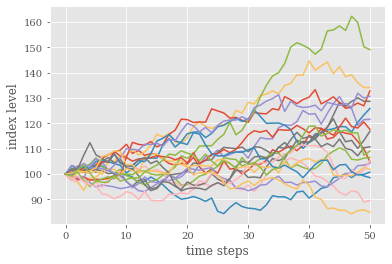📓

# The Black-Scholes-Merton model

In 1973, economists Fischer Black and Myron Scholes published the article “The Pricing of Options and Corporate Liabilities” in the Journal of Political Economy. Robert C. Merton published the article “Theory of Rational Option Pricing” in the Bell Journal of Economics and Management Science that same year. For their work, Merton and Scholes won the Nobel Memorial Prize in Economics in 1997. Black had died two years earlier unfortunately. The BSM model is now the basis of many option pricing models deployed in practice.

Black-Scholes Option Pricing example

One of the most important assumptions in the Black-Scholes model is that log gross returns are normally distributed with mean and variance that scales directly with time to expiration ((μ-σ²/2) * (T-t), σ² * (T-t)). This is sometimes termed the BSM model of (underlier) prices. Using the BSM model for the underlier, along with a risk-free rate, one can obtain a partial differential equation (PDE) for the evolution of (European) option prices. Solving the PDE gives us some useful expressions for pricing options. We exclude these details, but for those interested, please see the references below. After some manipulation, and a decent dose of solving PDEs, the European call price is given by:

where:

K is the strike price, r is the risk-free interest rate (usually per year), T-t is the time to maturity (usually in years), where t is the current date and T is the expiration date. S is today’s underlying price. σ is the volatility (also usually measured on an annual basis). N denotes the cumulative standard normal distribution function, with mean 0 and standard deviation 1. For example:

N(-1) = 0.1587, N(0) = 0.5, N(1) = 0.8413.

Using put-call parity (long call + short put = long underlying + short bond), and the result that

N(d) + N(-d) = 1 we can write:

for the corresponding price of a European put option.

Let’s try out the expression with some values to get a feel for what is going on (example modified from Jarrow Example 19.1).

Take S = 100, K = 110, = 1, t = 0, σ = 0.14247 per year, and r = 5 percent per year.

We compute d₁ = -0.2468 and d₂ = -0.3893, and

N(d₁) = 0.4025 and N(d₂) = 0.3485, and

exp(-r(T-t)) = exp(-0.05) = 0.9513 (this is interpreted as the zero-coupon bond price).

The call price is then:

c = S*N(d₁)-K*exp(-r(T-t))*N(d₂)

= 100*0.4025 -110*0.9513*0.3485 = \$3.78

If K is even higher than 110, then c decreases, since the option is more out-of-the-money, and vice-versa. The longer the time to expiration, the smaller the second term and consequently the higher the value of the option, reflecting the time decay. Using this formula, it can be shown (by taking the derivative of c with respect to σ) that as σ increases, so does the value of the call option. Intuitively, the higher the volatility, the more uncertainty in the future price of the underlying, and the greater the magnitude of the payoffs in the scenarios where the option expires in-the-money, resulting in a higher value of the call option. Similarly, the price of a put option also increases with volatility.

Volatility

There are two types of volatility that are discussed in the context of options: historical volatility and implied volatility. Historical volatility refers to how much the price of the underlying has fluctuated for each time interval (e.g. 1 day) on average, in the past, say 1 year. Implied volatility is the level of volatility of the underlying that is implied by the current option price under some assumptions about the option pricing model. We’ll discuss pricing models in future articles.

Assumptions of the BSM model

1. No market frictions (no transaction costs, taxes, continuous trading etc.)
2. No credit risk (so one can borrow or lend any amount at the risk-free interest rate)
3. Competitive markets (so one can buy and sell any amount of the asset without price impact)
4. No arbitrage opportunities (so no chance of making a profit without risk)
5. The risk-free interest rate is constant
6. No intermediate cash flows (i.e. no asset dividends or bond coupons — this can be accounted for)
7. The returns on the asset (continuously compounded) follow a normal distribution. We’ll elaborate more on this now:

More Details on Lognormally Distributed Prices

We previously saw how an L dollars in a continuously compounded risk-free account grows to L(T) = L(0)exp(rT) after time T where r is the risk-free rate We could analogously write S(T) = S(0)exp(γT), where γ is the continuously compounded rate of return on the underlying asset.

The problem with this expression is that it doesn’t take into account risk. Asset returns should be allowed to be positive, negative or zero to match what is observed in financial markets. The assumption made in the BSM model is that the return, γ, is normally distributed with mean (μ-σ²/2)(T-t) and standard deviation σ*sqrt(T-t), so we have that:

where z is a standard normal random variable with mean 0 and variance 1. Another way of saying this is that z has a probability density function given by: φ(z) = exp^(-z²/2)/sqrt(2π).

Notice also that if we divide both sides by S(t) and take a logarithm on both sides, we see that the gross returns are lognormally distributed (this is just saying that the logarithm of the return on the underlying, log(S(T)/S(t)), follows a normal distribution).

The following is a plot of the prices given by the above formula, with S = 100, = 1, σ = 0.14247 per year, and r = 5 percent per year. The year is broken into 50 time steps, and 20 paths are plotted.In our next article, we’ll discuss extensions to the Black-Scholes model and the circumstances in which the model does not hold well.

References

Jarrow, Chatterjea — An Introduction to Derivatives, Securities, Financial Markets, and Risk Management, Chapter 19